Courses

# Sorting MCQ - 2

## 20 Questions MCQ Test GATE Computer Science Engineering(CSE) 2022 Mock Test Series | Sorting MCQ - 2

Description
This mock test of Sorting MCQ - 2 for Computer Science Engineering (CSE) helps you for every Computer Science Engineering (CSE) entrance exam. This contains 20 Multiple Choice Questions for Computer Science Engineering (CSE) Sorting MCQ - 2 (mcq) to study with solutions a complete question bank. The solved questions answers in this Sorting MCQ - 2 quiz give you a good mix of easy questions and tough questions. Computer Science Engineering (CSE) students definitely take this Sorting MCQ - 2 exercise for a better result in the exam. You can find other Sorting MCQ - 2 extra questions, long questions & short questions for Computer Science Engineering (CSE) on EduRev as well by searching above.
QUESTION: 1

### The number of elements that can be sorted in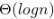time using heap sort is

Solution:

Time complexity of Heap Sort is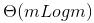for m input elements. For m =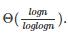, the value of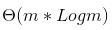will be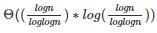which is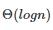QUESTION: 2

Solution:
QUESTION: 3

### Given an array where numbers are in range from 1 to n6, which sorting algorithm can be used to sort these number in linear time?

Solution:

See Radix Sort for explanation.

QUESTION: 4

In quick sort, for sorting n elements, the (n/4)th smallest element is selected as pivot using an O(n) time algorithm. What is the worst case time complexity of the quick sort?

Solution:

Answer(B) The recursion expression becomes: T(n) = T(n/4) + T(3n/4) + cn After solving the above recursion, we get heta(nLogn).

QUESTION: 5

Consider the Quicksort algorithm. Suppose there is a procedure for finding a pivot element which splits the list into two sub-lists each of which contains at least one-fifth of the elements. Let T(n) be the number of comparisons required to sort n elements. Then

Solution:

For the case where n/5 elements are in one subset, T(n/5) comparisons are needed for the first subset with n/5 elements, T(4n/5) is for the rest 4n/5 elements, and n is for finding the pivot. If there are more than n/5 elements in one set then other set will have less than 4n/5 elements and time complexity will be less than T(n/5) + T(4n/5) + n because recursion tree will be more balanced.

QUESTION: 6

Let P be a QuickSort Program to sort numbers in ascending order using the first element as pivot. Let t1 and t2 be the number of comparisons made by P for the inputs {1, 2, 3, 4, 5} and {4, 1, 5, 3, 2} respectively. Which one of the following holds?

Solution:

When first element or last element is chosen as pivot, Quick Sort's worst case occurs for the sorted arrays. In every step of quick sort, numbers are divided as per the following recurrence. T(n) = T(n-1) + O(n)

QUESTION: 7

You have an array of n elements. Suppose you implement quicksort by always choosing the central element of the array as the pivot. Then the tightest upper bound for the worst case performance is

Solution:

The central element may always be an extreme element, therefore time complexity in worst case becomes O(n2)

QUESTION: 8

In a permutation a1.....an of n distinct integers, an inversion is a pair (ai, aj) such that i < j and ai > aj. What would be the worst case time complexity of the Insertion Sort algorithm, if the inputs are restricted to permutations of 1.....n with at most n inversions?

Solution:

Insertion sort runs in Θ(n + f(n)) time, where f(n) denotes the number of inversion initially present in the array being sorted.

QUESTION: 9

Randomized quicksort is an extension of quicksort where the pivot is chosen randomly. What is the worst case complexity of sorting n numbers using randomized quicksort?

Solution:

Randomized quicksort has expected time complexity as O(nLogn), but worst case time complexity remains same. In worst case the randomized function can pick the index of corner element every time.

QUESTION: 10

Which of the following changes to typical QuickSort improves its performance on average and are generally done in practice.

1) Randomly picking up to make worst case less likely to occur.
2) Calling insertion sort for small sized arrays to reduce recursive calls.
3) QuickSort is tail recursive, so tail call optimizations can be done.
4) A linear time median searching algorithm is used to pick the median, so that the worst case time reduces to O(nLogn)

Solution:

The 4th optimization is generally not used, it reduces the worst case time complexity to O(nLogn), but the hidden constants are very high.

QUESTION: 11

Which one of the following is the recurrence equation for the worst case time complexity of the Quicksort algorithm for sorting n(≥ 2) numbers? In the recurrence equations given in the options below, c is a constant.

Solution:

In worst case, the chosen pivot is always placed at a corner position and recursive call is made for following. a) for subarray on left of pivot which is of size n-1 in worst case. b) for subarray on right of pivot which is of size 0 in worst case.

QUESTION: 12

Assume that a mergesort algorithm in the worst case takes 30 seconds for an input of size 64. Which of the following most closely approximates the maximum input size of a problem that can be solved in 6 minutes?

Solution:

Time complexity of merge sort is Θ(nLogn)

c*64Log64 is 30
c*64*6 is 30
c is 5/64

For time 6 minutes
5/64*nLogn = 6*60
nLogn = 72*64 = 512 * 9
n = 512.

QUESTION: 13

The worst case running times of Insertion sort, Merge sort and Quick sort, respectively, are:

Solution:
• Insertion Sort takes Θ(n2) in worst case as we need to run two loops. The outer loop is needed to one by one pick an element to be inserted at right position. Inner loop is used for two things, to find position of the element to be inserted and moving all sorted greater elements one position ahead. Therefore the worst case recursive formula is T(n) = T(n-1) + Θ(n).
• Merge Sort takes Θ(n Log n) time in all cases. We always divide array in two halves, sort the two halves and merge them. The recursive formula is T(n) = 2T(n/2) + Θ(n).
• QuickSort takes Θ(n2) in worst case. In QuickSort, we take an element as pivot and partition the array around it. In worst case, the picked element is always a corner element and recursive formula becomes T(n) = T(n-1) + Θ(n). An example scenario when worst case happens is, arrays is sorted and our code always picks a corner element as pivot.
QUESTION: 14

Assume that the algorithms considered here sort the input sequences in ascending order. If the input is already in ascending order, which of the following are TRUE?

I. Quicksort runs in Θ(n2) time
II. Bubblesort runs in Θ(n2) time
III. Mergesort runs in Θ(n) time
IV. Insertion sort runs in Θ(n) time

Solution:

I. Given an array in ascending order, Recurrence relation for total number of comparisons for quicksort will be T(n) = T(n-1)+O(n) //partition algo will take O(n) comparisons in any case. = O(n^2)
II. Bubble Sort runs in Θ(n^2) time If an array is in ascending order, we could make a small modification in Bubble Sort Inner for loop which is responsible for bubbling the kth largest element to the end in kth iteration. Whenever there is no swap after the completion of inner for loop of bubble sort in any iteration, we can declare that array is sorted in case of Bubble Sort taking O(n) time in Best Case.
III. Merge Sort runs in Θ(n) time Merge Sort relies on Divide and Conquer paradigm to sort an array and there is no such worst or best case input for merge sort. For any sequence, Time complexity will be given by following recurrence relation, T(n) = 2T(n/2) + Θ(n) // In-Place Merge algorithm will take Θ(n) due to copying an entire array. = Θ(nlogn)
IV. Insertion sort runs in Θ(n) time Whenever a new element which will be greater than all the elements of the intermediate sorted sub-array ( because given array is sorted) is added, there won't be any swap but a single comparison. In n-1 passes we will be having 0 swaps and n-1 comparisons. Total time complexity = O(n) // N-1 Comparisons

/// For an array already sorted in ascending order, Quicksort has a complexity Θ(n2) [Worst Case] Bubblesort has a complexity Θ(n) [Best Case] Mergesort has a complexity Θ(n log n) [Any Case] Insertsort has a complexity Θ(n) [Best Case]

QUESTION: 15

Assume that we use Bubble Sort to sort n distinct elements in ascending order. When does the best case of Bubble Sort occur?

Solution:
QUESTION: 16

If we use Radix Sort to sort n integers in the range (nk/2,nk], for some k>0 which is independent of n, the time taken would be?

Solution:

Radix sort time complexity = O(wn)
for n keys of word size= w
=>w = log(nk
O(wn)=O(klogn.n)
=> kO(nlogn)

QUESTION: 17

Consider an array of elements arr= {5,4,3,2,1} , what are the steps of insertions done while doing insertion sort in the array.

Solution:

In the insertion sort , just imagine that the first element is already sorted and all the right side Elements are unsorted, we need to insert all elements one by one from left to right in the sorted Array. Sorted : 5
unsorted : 4 3 2 1 Insert all elements less than 5 on the left (Considering 5 as the key ) Now key value is 4 and array will look like this Sorted : 45 unsorted : 3 2 1 Similarly for all the cases the key will always be the newly inserted value and all the values will be compared to that key and inserted in to proper position

QUESTION: 18

Which is the correct order of the following algorithms with respect to their time Complexity in the best case?

Solution:

In best case,

Quick sort: O (nlogn)
Merge sort: O (nlogn)
Insertion sort: O (n)
Selection sort: O (n^2)

QUESTION: 19

Which of the following statements is correct with respect to insertion sort?

*Online - can sort a list at runtime
*Stable - doesn't change the relative order of elements with equal keys.

Solution:

Time taken by algorithm is good for small number of elements, but increases quadratically for large number of elements.

QUESTION: 20

Consider the array A[]= {6,4,8,1,3} apply the insertion sort to sort the array . Consider the cost associated with each sort is 25 rupees , what is the total cost of the insertion sort when element 1 reaches the first position of the array?

Solution:

When the element 1 reaches the first position of the array two comparisons are only required hence 25 * 2= 50 rupees. *step 1: 4 6 8 1 3 . *step 2: 1 4 6 8 3.